# Leader Selection in Stochastically Forced Consensus Networks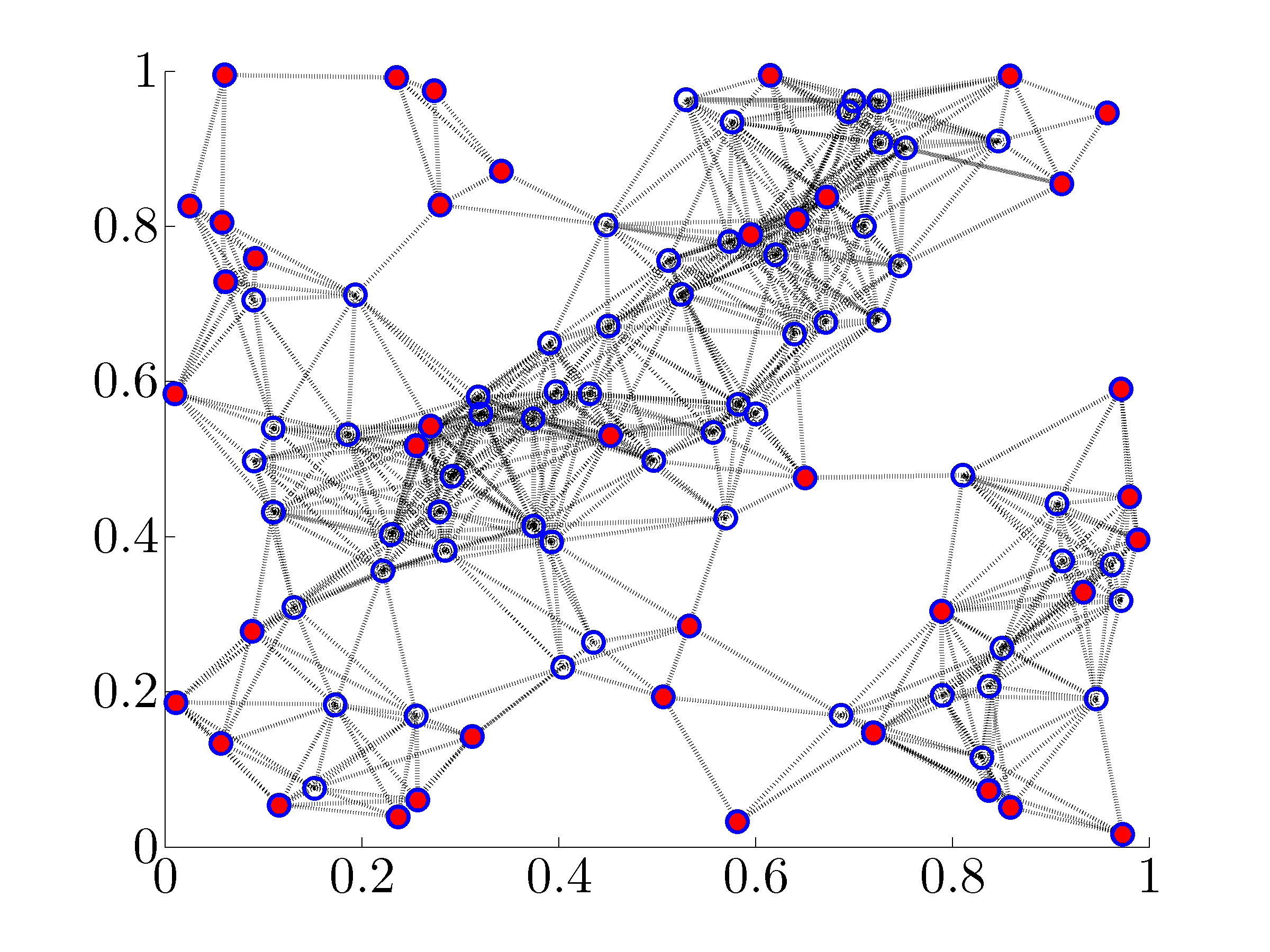June 2013 Matlab Files Download all Matlab files Presentation 2013 Allerton Talk

## Purpose

This website provides a Matlab implementation of algorithms that quantify suboptimality of solutions to two combinatorial network optimization problems. For undirected consensus networks, these problems arise in assignment of a pre-specified number of nodes, as leaders, in order to minimize the mean-square deviation from consensus. For distributed localization in sensor networks, one of our formulations is equivalent to the problem of choosing a pre-specified number of absolute position measurements among available sensors in order to minimize the variance of the estimation error.

## Two combinatorial problems involving graph Laplacian

### Diagonally strengthened graph Laplacian

Let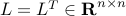be the graph Laplacian of an undirected connected network. A diagonally strengthened graph Laplacian is obtained by adding a vector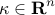with positive elements to the main diagonal of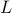,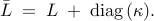For connected graphs, the diagonally strengthened Laplacian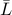is a positive definite matrix.

We are interested in selecting a pre-specified number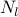of diagonal elements ofsuch that the trace of the inverse of the strengthened graph Laplacian is minimized. This problem can be expressed as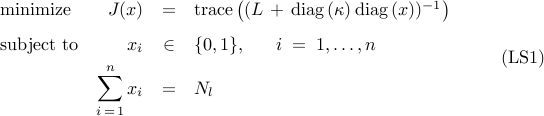where the graph Laplacianand the vector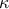with positive elements are problem data, and the Boolean-valued vector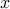with cardinalityis the optimization variable.

This combinatorial optimization problem is of interest in several applications. For example, in leader-follower networks subject to stochastic disturbances, the problem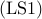amounts to assigningnodes as leaders (that have access to their own states) such that the mean-square deviation from consensus is minimized. We refer toas the noise-corrupted leader selection problem. Also, it can be shown that the problem of choosingabsolute position measurements among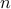sensors in order to minimize the variance of the estimation error in sensor networks is equivalent to the problem.

### Principal submatrices of graph Laplacian

Let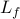be a principal submatrix of the graph Laplacianobtained by deleting a pre-specified numberof rows and columns. For undirected connected graphs,is a positive definite matrix.

The problem of finding a principal submatrixofsuch that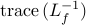is minimized can be expressed as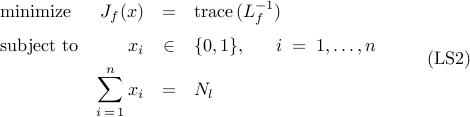where the Boolean-valued vectorwith cardinalityis the optimization variable. The index set of nonzero elements ofidentifies the rows and columns of the graph Laplacianthat need to be deleted in order to obtain.

Similar to, the combinatorial optimization problem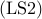arises in several applications. For example, in leader-follower networks, the problemamounts to assigningnodes as leaders (that perfectly follow their desired trajectories) such that the mean-square deviation from consensus is minimized. We refer toas the noise-free leader selection problem.

## Performance bounds on the global optimal value

For large graphs, it is challenging to determine global solutions to the combinatorial optimization problemsand. Instead, we develop efficient algorithms that quantify performance bounds on the global optimal values. Specifically, we obtain lower bounds by solving convex relaxations ofandand we obtain upper bounds using a simple but efficient greedy algorithm. We note that the greedy approach also provides feasible points ofand.

### Lower bounds from convex relaxations

Since the objective function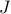inis the composition of a convex function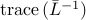of a positive definite matrixwith an affine function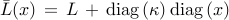, it follows thatis a convex function of. By enlarging the Boolean constraint set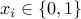to its convex hull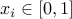, we obtain the following convex relaxation of: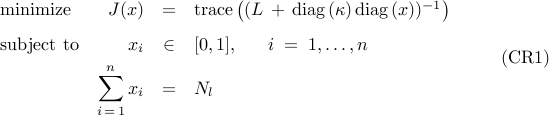Schur complement can be used to cast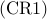as a semidefinite program (SDP). Thus,can be solved using general-purpose SDP solvers with computational complexity of order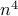. In contrast, computational complexity of a customized interior point method that we develop is of order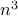.

In contrast to, the objective function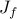inis a nonconvex function of. By a change of optimization variables, we identify the source of nonconvexity in the form of a rank constraint. Removal of the rank constraint and relaxation of the Boolean constraints can be used to obtain the following convex relaxation of: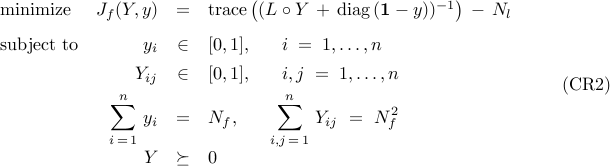Here, the symmetric matrix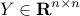and the vector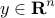are the optimization variables,is the elementwise multiplication of two matrices,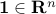is the vector of all ones, and the integer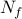is given by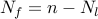.

Similar to, the convex relaxation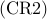can be cast as an SDP and solved using general-purpose SDP solvers. However, since this approach requires the computational complexity of order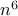, we develop an alternative approach that is well-suited for large problems. Our approach relies on the use of the alternating direction method of multipliers (ADMM) which allows us to decomposeinto a sequence of subproblems that can be solved with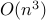operations.

### Upper bounds using a greedy algorithm

With lower bounds on the global optimal value resulting from the convex relaxationsand, we use a greedy algorithm to compute upper bounds. This algorithm selects one leader at a time by assigning the node that provides the largest performance improvement as the leader. Once this is done, an attempt to improve a selection ofleaders is made by checking possible swaps between the leaders and the followers. In both steps, substantial improvement in algorithmic complexity is achieved by exploiting structure of the low-rank modifications to Laplacian matrices.

Detailed description of the aforementioned algorithms (i.e., the greedy algorithm, the customized interior point method for, and the ADMM-based algorithm for) are provided in our paper

## Description of the main function

Matlab Syntax
```>> [Jlow,Jup,x] = leaders(L,Nl,kappa,flag);
```

Our Matlab function leaders.m takes the graph Laplacian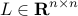, the positive integer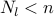, the vectorwith positive elements, and the indicator variable

•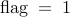–  for the noise-corrupted leader selection formulation;

•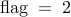–  for the noise-free leader selection formulation.

leaders.m returns the lower and upper bounds on the global optimal value of the leader selection problemor, along with the Boolean-valued vectorwhich represents a feasible point for the selection of leaders.

## Acknowledgements

This project is supported in part by the National Science Foundation under

• CAREER Award CMMI-0644793

• Award CMMI-0927509

• Award CMMI-0927720.